#### How to Calculate Percentile?

If you have written any competitive exams, you might be familiar with the term percentile. Most of today’s competitive entrance exams publish the results of the applicants based on percentiles. You might be familiar with the term percent. But, it is not the same as percentile.

Do not confuse these terms. As we all know, percentage is a number defined as a fraction of 100. So, if a person says that he scored 60% marks in his GMAT exam, then it means that if total score is 100, he scored 60 marks.

Obviously, if the total score is 50, the person has got 30 marks in the exam.

## What is Percentile?

A percentile is a measure at or below which a certain percentage of the distribution lies. So, if a person says he scored 60 percentile on his GMAT exam, then it means that 60 percent of the students scored equal to or lower than him and 40 percent of the students exceeded his score. With this, you cannot tell that the person scored 60 marks in a 100-mark exam.

The only information you get is that there are 59 students who scored less than your score and 40 students who scored more than you if the total number of students is 100.

In short, percentage gives us correct information about how an individual performed on a test, whereas with percentile, you cannot understand the actual score a person achieved. Percentile gives us information about how that score compares to the scores of other test takers.

Excel offers mainly four functions named PERCENTILE.INC, PERCENTILE.EXC, PERCENTRANK.INC and PERCENTRANK.EXC. Let’s calculate the relative percentile scores of 10 students. In that case, we can use either PERCENTRANK.INC or PERCENTRANK.EXC.

The PERCENTRANK.INC function will return the result as a number between 0 and 1 (both inclusive) and PERCENTRANK.EXC function will return the result as a number between 0 and 1 (both exclusive).

Open Excel and save the file as percentile.xlsx. Type “Name”, “Score” and “Percentile” in the cells A1, A2 and A3. You can format these cells to make them bold. Type any ten names in cells from A2 to A11 and enter any ten marks in cells from B2 to B11.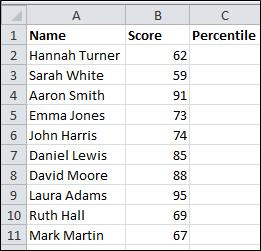## Syntax

Let’s start from checking the syntax of PERCENTRANK.INC function which will let us to calculate a percentile.

=PERCENTRANK.INC (data range, kth percentile)

• data range: An array or cell range of numeric values
• kth percentile: A percentile value between 0 and 1.

## Calculate Percentile

Click cell C2 and go to Formulas (main menu) –> More Functions (in the Function Library group) –> Statistical and select PERCENTRANK.INC function.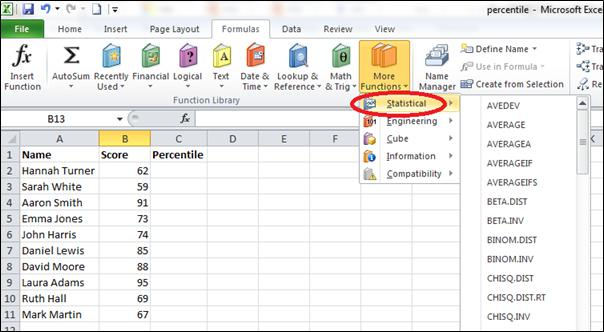You will get a window like this: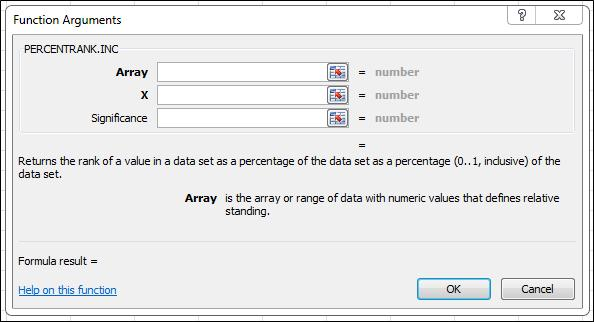In the textbox next to Array, enter “B\$2:B\$11” (without double quotes). Enter B2 in the textbox next to X and enter 1 in the textbox next to Significance.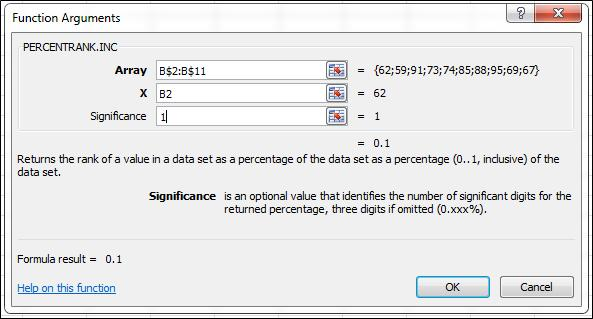In the formula bar, enter “*100” (without double quotes) next to the formula.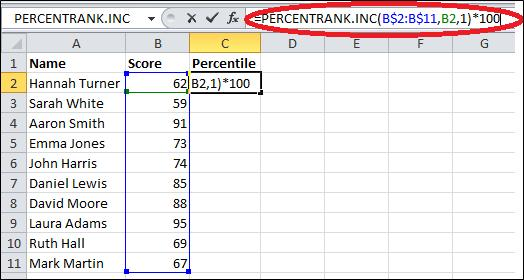Now copy the formula in cell C1 and paste the same in cells C2 to C11.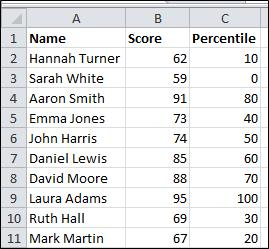If you analyze the data, you could find that the percentile of Sarah White is 0 and the percentile of Laura Adams is 100. If you analyze the scores of all the ten students, you will see that Sarah White has the lowest score and Laura Adams has the highest score.

The percentile of John Harris is 50, which means that 50% of the students (5 out of 10 students) have scored less than John Harris. The students who scored less than John Harris are Hannah Turner, Sarah White, Emma Jones, Ruth Hall, and Mark Martin (a total of 5 students).

If you sort the data by scores, you will get a clearer picture. Copy the whole dataset (cells A1 through C11) and paste it in cells from F1 through H11 in the same worksheet.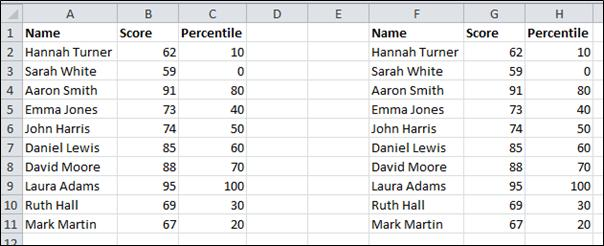Select the newly copied data and go to Data (main menu) > Sort (in the Sort & Filter) section and click Sort.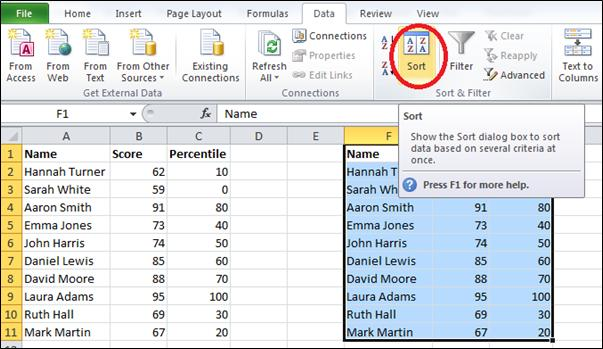You will get a window like this: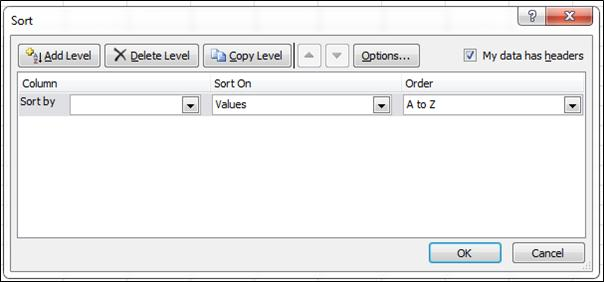From the Sort by list, select Score and from the Order list, select Largest to Smallest.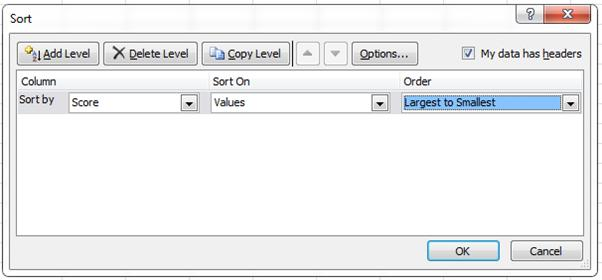Click OK and now your data will be like this: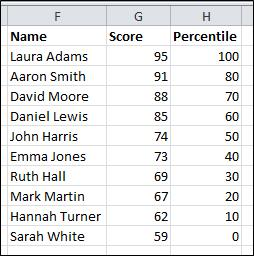Now you can analyze the data easily.

## Using PERCENTILE.INC and PERCENTILE.EXC functions

Here’s how you can use the PERCENTILE.EXC and PERCENTILE.INC functions to calculate percentiles in Excel:

PERCENTILE.EXC Function: The PERCENTILE.EXC function calculates the percentile excluding the boundary values.

The syntax of the function is:

=PERCENTILE.EXC(array, k)

Where:

• Array: It is the range of cells that contains the data for which you want to calculate the percentile.
• k: It is the percentile value in decimal form.

Example: Suppose you have data in cells A1 to A10, and you want to find the 75th percentile using the PERCENTILE.EXC function. The formula would be: =PERCENTILE.EXC(A1:A10,0.75)

PERCENTILE.INC Function: The PERCENTILE.INC function calculates the percentile including the boundary values.

The syntax of the function is:

=PERCENTILE.INC(array, k)

Where:

• Array: It is the range of cells that contains the data for which you want to calculate the percentile.
• k: It is the percentile value in decimal form.

Example: Suppose you have data in cells A1 to A10, and you want to find the 75th percentile using the PERCENTILE.INC function. The formula would be: =PERCENTILE.INC(A1:A10,0.75)

Note: The difference between the PERCENTILE.EXC and PERCENTILE.INC functions is in how they treat the boundary values. The PERCENTILE.EXC function excludes the boundary values while the PERCENTILE.INC function includes them.

## Key Takeaways

• Percentile is a measure at or below which a certain percentage of the distribution lies.
• The PERCENTILE.INC and PERCENTILE.EXC functions in Excel can be used to calculate percentiles.
• The PERCENTILE.INC function calculates the percentile including the boundary values, while the PERCENTILE.EXC function excludes the boundary values.

## FAQ

• Q: What is the difference between percentile and percentage?
• A: Percentile is a measure of how a particular value compares to the rest of the values in a group, while percentage is a measure of how much a particular value is out of 100.
• Q: How do I use the PERCENTILE.INC and PERCENTILE.EXC functions in Excel?
• A: The syntax for the PERCENTILE.INC function is =PERCENTILE.INC(array, k), where array is the range of cells that contains the data for which you want to calculate the percentile, and k is the percentile value in decimal form. The syntax for the PERCENTILE.EXC function is =PERCENTILE.EXC(array, k).